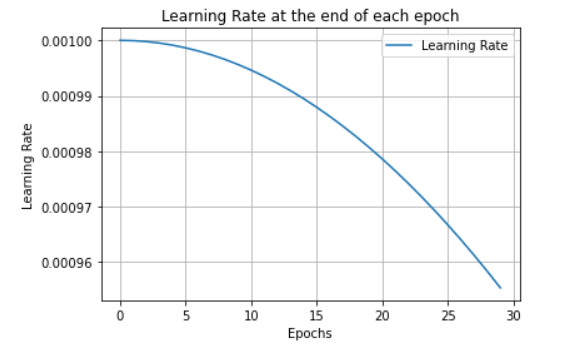# How to fix my learning rateI am using cosine annealing scheduler, but it is adjusting and such a small rate.

This is how it is initialized:

lrs = torch.optim.lr_scheduler.CosineAnnealingLR(optimizer, T_max = len(train_loader))

and this is how it is being called:

```````for i in range(epoch):`

`trn_corr = 0`

`tst_corr = 0`

`#lrs.step()`

`#adjust the learning rate after 30 epochs`

`#Run training for one epoch`

`#evaluate the validation/test`

`lrs.step()`
``````

inside the training loop the optimizer is being adjusted like so:

```````# Update parameters`

You can call `lrs.step()` for each iteration (or after a certain number of itertations) instead of once per epoch.
You would have to call `lrs.step()` inside the `DataLoader` loop, which is most likely used inside your `train` method.
In my other post the `range(nb_batches)` loop would correspond to the `DataLoader` loop.